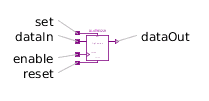Potential Sensor - MapleSim Help

Quasistationary Singlephase Potential Sensor

Potential sensorDescription The Quasistationary Singlephase Potential Sensor (or Potential Sensor) component measures the complex potential at the pin.Equations $i=0$ $y=v$ $\mathrm{\omega }=\stackrel{.}{\mathrm{\gamma }}$Variables

 Name Units Description Modelica ID $\mathrm{\omega }$ $\frac{\mathrm{rad}}{s}$ Angular frequency omegaConnections

 Name Description Modelica ID $\mathrm{pin}$ Pin pin $y$ Complex output; potential in $V$ yModelica Standard Library The component described in this topic is from the Modelica Standard Library. To view the original documentation, which includes author and copyright information, click here.